# 21 Results

View
Selected filters:
• MCCRS.Math.Content.6.NS.C.8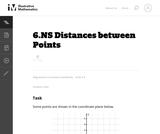Unrestricted Use
CC BY
Rating

This is a task from the Illustrative Mathematics website that is one part of a complete illustration of the standard to which it is aligned. Each task has at least one solution and some commentary that addresses important asects of the task and its potential use. Here are the first few lines of the commentary for this task: Some points are shown in the coordinate plane below. What is the distance between points B & C? What is the distance between points D & B? What is the ...

Subject:
Mathematics
Material Type:
Activity/Lab
Provider:
Illustrative Mathematics
Provider Set:
Illustrative Mathematics
Author:
Illustrative Mathematics
09/06/2013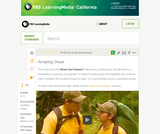Educational Use
Rating

This video from WOUB Athens, presented as a parody of a popular TV network reality show, will help high school students review and prepare for the Ohio Graduation Test in Mathematics.

Subject:
Mathematics
Material Type:
Lecture
Provider:
Ohio Digital Classroom
PBS LearningMedia
Provider Set:
Teachers' Domain
Author:
Ohio Digital Classroom
WOUB
09/16/2008
Remix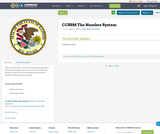Conditional Remix & Share Permitted
CC BY-SA
Rating

This resource links to both the Fractions progression document published by the Common Core Writing Teams in June 2011 and the Module posted on the Illustrative Mathematics website.

Material Type:
Assessment
Homework/Assignment
Teaching/Learning Strategy
Author:
ISBE Math Specialists
08/08/2019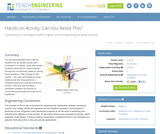Educational Use
Rating

This lab demonstrates Ohm's law as students set up simple circuits each composed of a battery, lamp and resistor. Students calculate the current flowing through the circuits they create by solving linear equations. After solving for the current, I, for each set resistance value, students plot the three points on a Cartesian plane and note the line that is formed. They also see the direct correlation between the amount of current flowing through the lamp and its brightness.

Subject:
Engineering
Physics
Material Type:
Activity/Lab
Provider:
TeachEngineering
Provider Set:
TeachEngineering
Author:
Aubrey McKelvey
09/18/2014Educational Use
Rating

In this Cyberchase video, Inez and Digit identify the location of the transformation using their knowledge of parallel and intersecting lines.

Subject:
Mathematics
Geometry
Material Type:
Lecture
Provider:
PBS LearningMedia
Provider Set:
PBS Learning Media Common Core Collection
Teachers' Domain
Author:
U.S. Department of Education
WNET
07/10/2008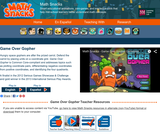Rating

Game Over Gopher is an exciting tower defense game that guides students in plotting coordinate pairs, differentiating negative coordinates from positive coordinates, and identifying the four quadrants. Hungry space gophers are marching towards a prize carrot, and to defend it players place tools around the coordinate grid to “feed” gophers and make them lose interest. Ruby mines (which must be placed at designated x, y coordinates) yield currency that players spend to strategically place carrot launchers, garlic rays, corn silos, and beet traps – gopher feeding tools. To introduce students to the coordinate grid, Game Over Gopher introduces new skills via interactive tutorials and uses consistent visual clues (e.g., x red, y blue) to guide players in plotting coordinates. This means it’s not necessary to teach students coordinate plotting ahead of time. As the levels progress, the number of tools available increases, the level of math vocabulary increases, the scale of the grid changes, and players are asked to expand their mastery of the grid by reflecting points across axes. The game lowers intimidation about the coordinate grid, helps students understand how positive and negative numbers reflect each other across the axes, and helps students get comfortable with the four quadrants.

Subject:
Mathematics
Material Type:
Game
Interactive
Provider:
Learning Games Lab
Author:
NMSU Learning Games Lab
07/20/2015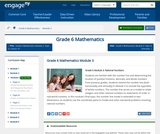Conditional Remix & Share Permitted
CC BY-NC-SA
Rating

Students are familiar with the number line and determining the location of positive fractions, decimals, and whole numbers from previous grades. Students extend the number line (both horizontally and vertically) in Module 3 to include the opposites of whole numbers. The number line serves as a model to relate integers and other rational numbers to statements of order in real-world contexts. In this module's final topic, the number line model is extended to two-dimensions, as students use the coordinate plane to model and solve real-world problems involving rational numbers.

Subject:
Numbers and Operations
Material Type:
Module
Provider:
New York State Education Department
Provider Set:
EngageNY
10/20/2013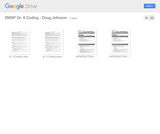Conditional Remix & Share Permitted
CC BY-NC
Rating

Students will develop basic skills in programming by using loops, control structures, and variables. Students will use the Tynker platform.

Subject:
Computer Science
Material Type:
Activity/Lab
Lesson Plan
Provider:
South Metro-Salem STEM Partnership
Author:
Doug Johnson
07/02/2015Conditional Remix & Share Permitted
CC BY-NC
Rating

Subject:
Mathematics
Material Type:
Full Course
Provider:
Pearson
10/06/2016Rating

Putting Math to Work

Type of Unit: Problem Solving

Prior Knowledge

Students should be able to:

Solve problems with rational numbers using all four operations.
Write ratios and rates.
Use a rate table to solve problems.
Write and solve proportions.
Use multiple representations (e.g., tables, graphs, and equations) to display data.
Identify the variables in a problem situation (i.e., dependent and independent variables).
Write formulas to show the relationship between two variables, and use these formulas to solve for a problem situation.
Draw and interpret graphs that show the relationship between two variables.
Describe graphs that show proportional relationships, and use these graphs to make predictions.
Interpret word problems, and organize information.
Graph in all quadrants of the coordinate plane.

Lesson Flow

As a class, students use problem-solving steps to work through a problem about lightning. In the next lesson, they use the same problem-solving steps to solve a similar problem about lightning. The lightning problems use both rational numbers and rates. Students then choose a topic for a math project. Next, they solve two problems about gummy bears using the problem-solving steps. They then have 3 days of Gallery problems to test their problem-solving skills solo or with a partner. Encourage students to work on at least one problem individually so they can better prepare for a testing situation. The unit ends with project presentations and a short unit test.

Subject:
Mathematics
Material Type:
Unit of Study
Provider:
PearsonConditional Remix & Share Permitted
CC BY-NC
Rating

Students work in a whole-class setting, independently, and with partners to design and implement a problem-solving plan based on the mathematical concepts of rates and multiple representations (e.g., tables, equations, and graphs). They analyze a rule of thumb and use this relationship to calculate the distance in miles from a viewer's vantage point to lightning.Key ConceptsThroughout this unit, students are encouraged to apply the mathematical concepts they have learned over the course of this year to new settings. Help students develop and refine these problem-solving skills:Creating a problem-solving plan and implementing the plan systematicallyPersevering through challenging problems to find solutionsRecalling prior knowledge and applying that knowledge to new situationsMaking connections between previous learning and real-world problemsCommunicating their approaches with precision and articulating why their strategies and solutions are reasonableCreating efficacy and confidence in solving challenging problems in the real worldGoals and Learning ObjectivesCreate and implement a problem-solving plan.Organize and interpret data presented in a problem situation.Analyze the relationship between two variables.Create a rate table to organize data and make predictions.Apply the relationship between the variables to write a mathematical formula and use the formula to solve problems.Create a graph to display proportional relationships, and use this graph to make predictions.Articulate strategies, thought processes, and approaches to solving a problem, and defend why the solution is reasonable.

Subject:
Algebra
Material Type:
Lesson Plan
Provider:
Pearson
09/21/2015Conditional Remix & Share Permitted
CC BY-NC
Rating

During this two-day lesson, students work with a partner to create and implement a problem-solving plan based on the mathematical concepts of rates, ratios, and proportionality. Students analyze the relationship between different-sized gummy bears to solve problems involving size and price.Key ConceptsThroughout this unit, students are encouraged to apply the mathematical concepts they have learned over the course of this year to new settings. Help students develop and refine these problem-solving skills:Creating a problem solving plan and implementing their plan systematicallyPersevering through challenging problems to find solutionsRecalling prior knowledge and applying that knowledge to new situationsMaking connections between previous learning and real-world problemsCommunicating their approaches with precision and articulating why their strategies and solutions are reasonableCreating efficacy and confidence in solving challenging problems in a real worldGoals and Learning ObjectivesCreate and implement a problem-solving plan.Organize and interpret data presented in a problem situation.Analyze the relationship between two variables.Use ratios.Write and solve proportions.Create rate tables to organize data and make predictions.Use multiple representations—including tables, graphs, and equations—to organize and communicate data.Articulate strategies, thought processes, and approaches to solving a problem, and defend why the solution is reasonable.

Subject:
Ratios and Proportions
Material Type:
Lesson Plan
Provider:
Pearson
09/21/2015Conditional Remix & Share Permitted
CC BY-NC
Rating

During this two-day lesson, students work with a partner to create and implement a problem-solving plan based on the mathematical concepts of rates, ratios, and proportionality. Students analyze the relationship between different-sized gummy bears to solve problems involving size and price.Key ConceptsThroughout this unit, students are encouraged to apply the mathematical concepts they have learned over the course of this year to new settings. Helping students develop and refine these problem solving skills:Creating a problem solving plan and implementing their plan systematicallyPersevering through challenging problems to find solutionsRecalling prior knowledge and applying that knowledge to new situationsMaking connections between previous learning and real-world problemsCommunicating their approaches with precision and articulating why their strategies and solutions are reasonableCreating efficacy and confidence in solving challenging problems in a real worldGoals and Learning ObjectivesCreate and implement a problem-solving plan.Organize and interpret data presented in a problem situation.Analyze the relationship between two variables.Use ratios.Write and solve proportions.Create rate tables to organize data and make predictionsUse multiple representations—including tables, graphs, and equations—to organize and communicate data.Articulate strategies, thought processes, and approaches to solving a problem and defend why the solution is reasonable.

Subject:
Ratios and Proportions
Material Type:
Lesson Plan
Provider:
Pearson
09/21/2015Conditional Remix & Share Permitted
CC BY-NC
Rating

Students create and implement a problem-solving plan to solve another problem involving the relationship between the sound of thunder and the distance of the lightning.Key ConceptsThroughout this unit, students are encouraged to apply the mathematical concepts they have learned over the course of this year to new settings. Help students develop and refine these problem-solving skills:Creating a problem-solving plan and implementing their plan systematicallyPersevering through challenging problems to find solutionsRecalling prior knowledge and applying that knowledge to new situationsMaking connections between previous learning and real-world problemsCommunicating their approaches with precision and articulating why their strategies and solutions are reasonableCreating efficacy and confidence in solving challenging problems in a real worldGoals and Learning ObjectivesCreate and implement a problem-solving plan.Organize and interpret data presented in a problem situation.Analyze the relationship between two variables.Create a rate table to organize data and make predictions.Apply the relationship between the variables to write a mathematical formula and use the formula to solve problems.Create a graph to display proportional relationships and use this graph to make predictions.Articulate strategies, thought processes, and approaches to solving a problem and defend why the solution is reasonable.

Subject:
Mathematics
Material Type:
Lesson Plan
Provider:
Pearson
09/21/2015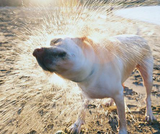Rating

Rational Numbers

Type of Unit: Concept

Prior Knowledge

Students should be able to:

Solve problems with positive rational numbers.
Plot positive rational numbers on a number line.
Understand the equal sign.
Use the greater than and less than symbols with positive numbers (not variables) and understand their relative positions on a number line.
Recognize the first quadrant of the coordinate plane.

Lesson Flow

The first part of this unit builds on the prerequisite skills needed to develop the concept of negative numbers, the opposites of numbers, and absolute value. The unit starts with a real-world application that uses negative numbers so that students understand the need for them. The unit then introduces the idea of the opposite of a number and its absolute value and compares the difference in the definitions. The number line and positions of numbers on the number line is at the heart of the unit, including comparing positions with less than or greater than symbols.

The second part of the unit deals with the coordinate plane and extends student knowledge to all four quadrants. Students graph geometric figures on the coordinate plane and do initial calculations of distances that are a straight line. Students conclude the unit by investigating the reflections of figures across the x- and y-axes on the coordinate plane.

Subject:
Mathematics
Numbers and Operations
Material Type:
Unit of Study
Provider:
PearsonConditional Remix & Share Permitted
CC BY-NC
Rating

Students draw a figure on the coordinate plane that matches a written description.Key ConceptsOrdered pairs name locations on the coordinate plane. The first coordinate tells how many units to go left or right of the origin (0,0) along the x-axis. The second coordinate tells how many units to go up or down from the origin along the y-axis.Goals and Learning ObjectivesDraw a figure that matches a description of a figure on the coordinate plane.Give coordinates of points on the coordinate plane.Write descriptions of figures on the coordinate plane.

Subject:
Numbers and Operations
Material Type:
Lesson Plan
Provider:
Pearson
09/21/2015Conditional Remix & Share Permitted
CC BY-NC
Rating

Students reflect a figure across one of the axes on the coordinate plane and name the vertices of the reflection. As they are working, students look for and make use of structure to identify a convention for naming the coordinates of the reflected figure.Key ConceptsWhen point (m,n) is reflected across the y-axis, the reflected point is (−m,n).When point (m,n) is reflected across the x-axis, the reflected point is (m,−n).When point (m,n) is reflected across the origin (0,0), the reflected point is (−m,−n).Goals and Learning ObjectivesReflect a figure across one of the axes on the coordinate plane.Name the vertices of the reflected figure.Discern a pattern in the coordinates of the reflected figure.

Subject:
Numbers and Operations
Material Type:
Lesson Plan
Provider:
Pearson
09/21/2015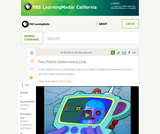Educational Use
Rating

In this video segment from Cyberchase, Digit must a make a straight line between the two points and then follow the path created.

Subject:
Mathematics
Geometry
Material Type:
Lecture
Provider:
PBS LearningMedia
Provider Set:
PBS Learning Media Common Core Collection
Teachers' Domain
Author:
U.S. Department of Education
WNET
07/10/2008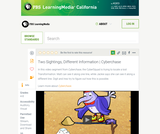Educational Use
Rating

Jackie and Matt are looking at the same object along two different lines, in this video segment from Cyberchase.

Subject:
Mathematics
Geometry
Material Type:
Lecture
Provider:
PBS LearningMedia
Provider Set:
PBS Learning Media Common Core Collection
Teachers' Domain
Author:
U.S. Department of Education
WNET
07/10/2008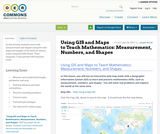Conditional Remix & Share Permitted
CC BY-NC-SA
Rating

In this activity, students work with measurement and shapes using live web maps and images of the Earth at various scales using ArcGIS Online. Time required: 2 class periods (100 minutes total).

Subject:
Career and Technical Education
Mathematics
Social Science
Material Type:
Lesson
Author:
Joseph Kerski